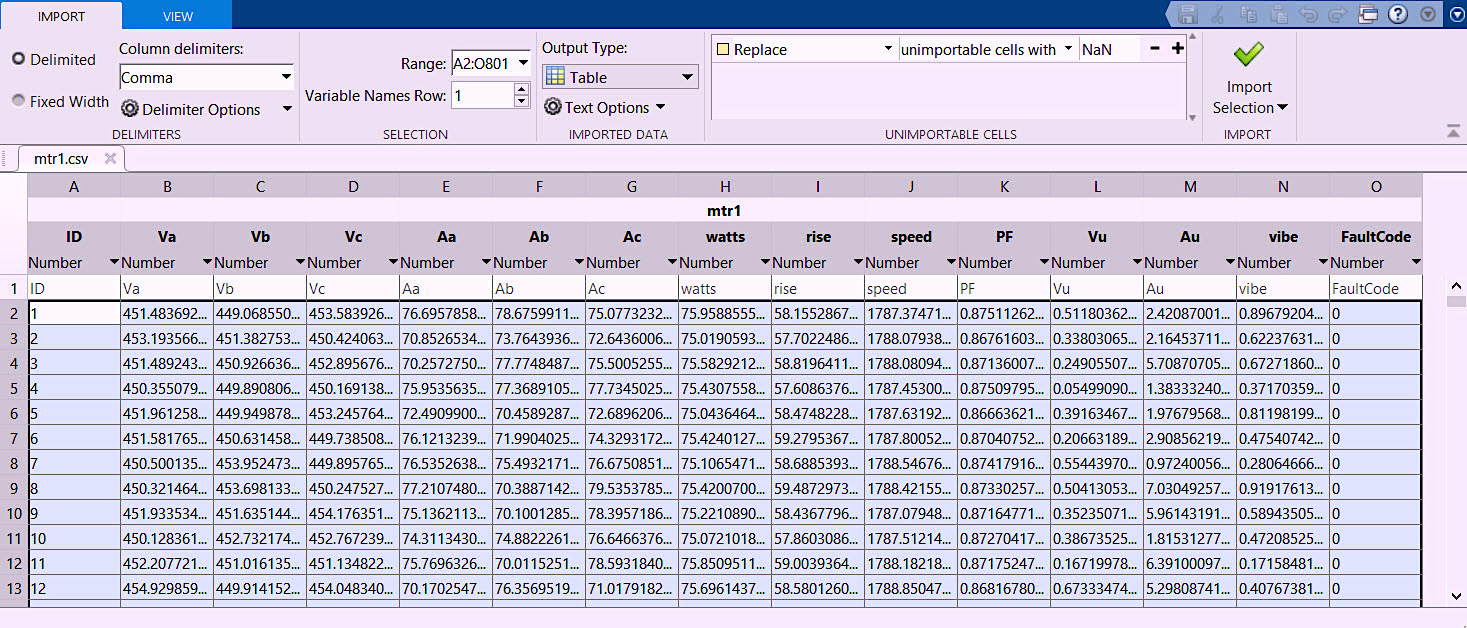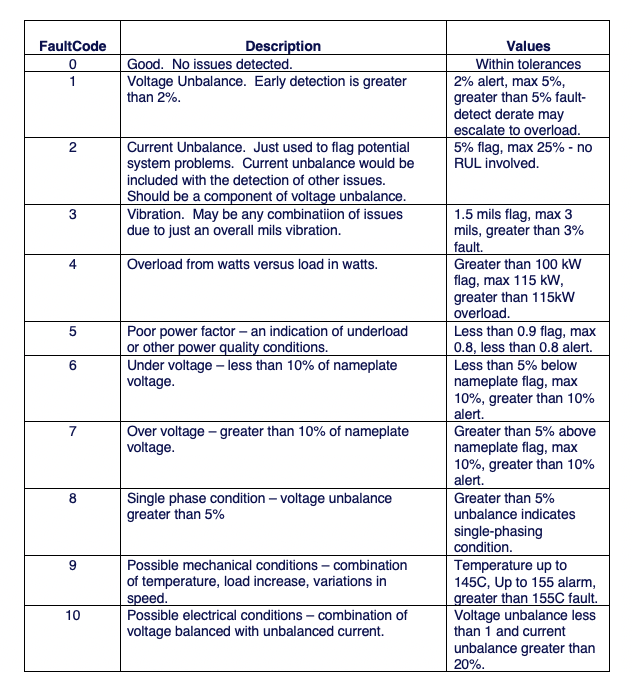Select Page

In Parts I and II of this particular series (see links below), we discussed using raw motor data for Machine Learning (ML); identified an example electric motor; selected an ML language; and explored some available motor data. Now we need to determine what we will be able to detect with that data and then gather some of it to train an ML algorithm to classify and provide information on Remaining Useful Life (RUL). While doing so requires us to actually have some data, in many cases, what we need may not be available. That means we must create it in some way, which can include generating a simulation, collecting data on an existing machine, and/or producing data based upon the curves we explored in Part II.

In this article, we discuss how to produce a number of comma delimited files (*.csv – with * representing any file name) using a Matlab script that we generate (see Sidebar at the end of this article). The code we generate for this demonstration will be relatively straightforward, with a constant load and speed. We will consider this data as being collected in one-hour increments.  Note: There are variations and noise introduced into the data. This is to avoid a condition referred to as “over-fitting,: in which the training data is so exact that any variation will throw false positives. The output of the script we’ve created will look similar that shown in Fig. 1.

Part I  (Aug. 8, 2021)

Part II (Aug. 15, 2021)Fig. 1. Snapshot of one *.csv file used for the ML project.

In Fig. 1, the variable “ID” is the hour increment. Then we have three voltages, three currents, the load in watts, the operating temperature in degrees C, the operating speed, power factor, voltage and current unbalance, vibration in mils, and something referred to as the “FaultCode.” The FaultCode variable will be used to set up simulated failure data and would be introduced with either point where the fault is detectible within the *.csv file.

For this example, we’re using FaultCode “0” to represent a good condition and will introduce additional numbers based upon the types of conditions we believe can be found with the provided data and combinations. The reason for this is, in part, to use a numerical fault code is if the end solution will be converted from a script to a function for conversion to a Python package for distribution. While scripts and functions are similar, there is a very important difference: A script is a procedure, or program, to perform a series of commands within the Matlab environment, whereas a function is specifically designed to manage input and output data.

As we review the data outlined within Fig. 1, we can determine types of problems that can be detected (see Table I below).Table I. Some Detectable Faults.

While more defects than those listed in Table I can be determined from our dataset, for the purposes of this article, we will limit them to these 10 conditions. For each of them, we can generate additional datasets for training by modifying the dataset code and creating sets based around progression towards the values identified in Table 1.

For some applications you can create a model within Matlab in a module called Simulink, which requires a reasonable skill level in programming.  As an aside, such models can be harnessed to generate useful data, as well as provide continuous flows of it for testing the algorithms being developed. (Note: We won’t be covering that aspect of model development in this article series, however.)

In our next article, we’ll discuss how to process the data we’ve generated and determine the next steps for fault classification and RUL.TRR

SIDEBAR: Sample Code for Creating Comma-Delimited Files in Matlab
For those wishing to explore the method described in this article, Mathworks, provides 30-day trials of its personal and business versions of the Matlab and Simulink systems. The *.csv files created for this article series (as shown in Fig. 1) were developed within Matlab. The resulting code (or script) can be downloaded at the following link: